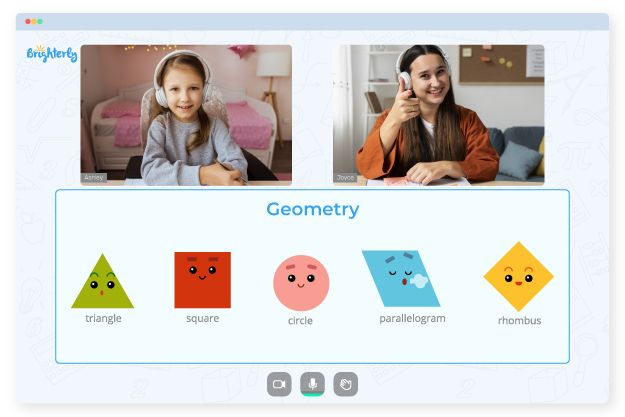The perimeter of a shape is the measurement of the length of the figure’s outline. It also measures the distance around the outside of a form or object. An analogy about walking around a body or building a fence around a field may help children to visualize this new term and understand the perimeters of shapes and the ways to measure perimeter.

Children might be given the perimeter of a shape and asked to work out the size of unlabeled edges. With the information provided in the perimeter worksheets grade 3, students can handle these questions easier and improve their geometry skills.

Calculating the perimeter in a perimeter worksheet 3rd grade would depend on your shape. However, if you have a form with straight sides, you can follow two simple steps: first, find the length of each edge of the shape or space. Once you’ve seen the measurements, you sum the lengths of all sides to find the perimeter.

Math for Kids

Is Your Child Struggling With Math?
1:1 Online Math Tutoring## Benefits of Perimeter 3rd Grade Worksheets

The best 3rd grade perimeter worksheets provide students with a step-by-step approach to solving problems on the topic and assist them in mastering the concept, beginning with finding the perimeter using grids. Kids will learn to determine the boundary of 2D shapes like squares, rectangles, triangles, trapezoids, rhombuses, kites, quadrilaterals, polygons, and circles. The worksheets provide a complete package, which makes them go-to tools.### Perimeter Worksheets 3rd Grade PDF### Perimeter Worksheets 3rd Grade PDF### Perimeter Worksheets 3rd Grade PDF### Perimeter Worksheets 3rd Grade PDF

A helpful way of introducing 3rd grade perimeter worksheets exercises is to ask children to think about walking around the sides of a shape as if it were a park. The analogy makes it easier to visualize that the perimeter is the total distance that they have walked. Teachers may then give children examples from the worksheets and ask them to add all the measurements to find the answer.

### Area and Perimeter Worksheets

Trouble with Geometry?• Is your child struggling to master the basics of geometry?
• Consider studying with an online tutor.

Does your child find understanding geometry to be challenging? Start lessons with an online tutor.